# How to do Percent Change Formula in Microsoft Excel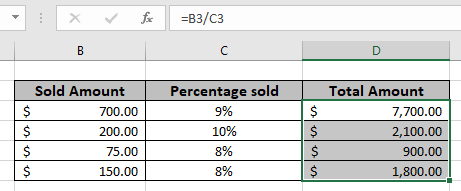In this article, we will learn about how to find the total amount from the percentage value in Excel.

Scenario :

For instance, working with percentage values in excel sometimes we need to get the total amount from the given percentage and the number. Given a discounted amount and discount percent, get the amount before discount or the total amount.

Apply percentage format

Convert 0.2 to 20% in Excel. Select the cell and Go to the % button under Cell formatting options shown below.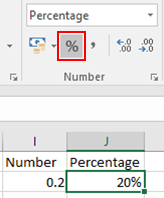Excel understands 0.20 equals to 20% in Excel.

Or you can right click the cell and select Format Cells… and a dialog box will appear as shown below.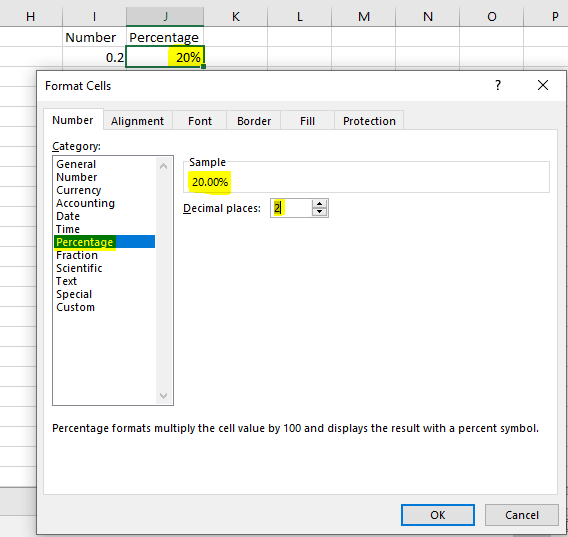Select the Percentage option from category and customize as required which can be seen as sample.

How to get the total amount?

Here we will use the basic mathematics observation used in excel. Excel considers percent value as decimal means 20% is 0.2 in excel. So we just need to divide the amount with percent value so as to get the total amount.

Generic formula:

 Total amount = discounted amount / discount percent

Discounted amount : amount after the discount applied

Discount percent : percent at which discount is given

Example:

All of these might be confusing to understand. So, let's test this formula via running it on the example shown below. Here we will use the generic formula to get the total value for the data.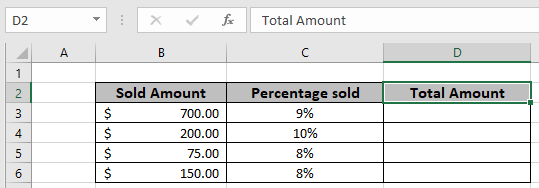Use the Formula in D3 cell

 = B3 / C3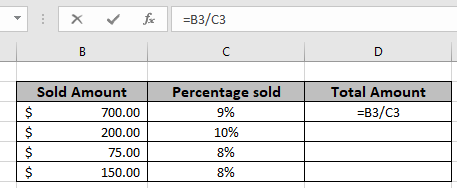The formula will be like as shown in the image above. Here the values to the function are given as cell reference.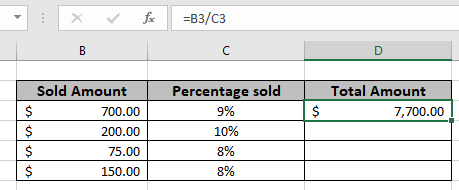As you can see in the above snapshot first old Amount comes out to be \$ 7,700. Copy the formula in the remaining cells using Ctrl + D shortcut, to get the New Amount for the rest of the values in the table.In the above snapshot the mathematical formula is used to get the total value from the percent amount in excel.

Notes :

1. The function returns #VALUE error if any of the argument is non numeric.
2. The function returns values for the negative as well as positive values.
3. Use the Increase by percentage formula to get the new or updated amount having percentage increase values.

Hope you understood How to do Percent Change Formula in Microsoft Excel. Explore more articles on Mathematical formulation in Excel here. If you liked our blogs, share it with your friends on Facebook. And also you can follow us on Twitter and Facebook. We would love to hear from you, do let us know how we can improve, complement or innovate our work and make it better for you. Write us at info@exceltip.com.

Related Articles:

Calculate Percentage of Total in Excel : Calculating percentages is a basic task in any field of work. We always want to know how much work is done. In school/collage we always calculated our attendance percentage carefully to be able to appear in the exam.

How to Calculate Percentage Discount in Excel : To calculate change in percentage discount of items, we simply subtract the discount price from total price and then get the percentage. This simple excel formula will get this done.

How to Increase by percentage in Excel : To increase a given value by a given percentage we can simply add the percentage of increment in 100% and then multiply the actual number with it. This will increase the given value with the given percentage.

How to Calculate Profit margin percentage in Excel : To calculate the profit margin we simply divide the sales price by purchase price and subtract the result from 1. This gives us the percentage of profit margin.

Mathematical Percentage Decrease Change in Excel : In this article, how to use percentage decrease change formula in Excel 2016.We need to find the percentage decrease in value due to decrement percentage change.

How to Calculate Project Complete Percentage in Excel : When each task is completed, we say that project is completed 100%. In that case we would  like to monitor how the task is completed while we work on the project. In this article, we will learn how you can calculate the project complete percentage in Excel

Get the percentage for the sold product in Excel : In this article, we will learn about how to calculate the percent for the sold product in excel. Get the Percent for the sold product data in excel using a simple formula. Divide the sold product amount by the total amount of the product.

Find Total Amount From Percentage : For instance, working with percentage values in excel sometimes we need to get the total amount from the given percentage and the number. Given a discounted amount and discount percent, get the amount before discount or the total amount.

Popular Articles :

How to use the IF Function in Excel : The IF statement in Excel checks the condition and returns a specific value if the condition is TRUE or returns another specific value if FALSE.

How to use the VLOOKUP Function in Excel : This is one of the most used and popular functions of excel that is used to lookup value from different ranges and sheets.

How to Use SUMIF Function in Excel : This is another dashboard essential function. This helps you sum up values on specific conditions.

How to use the COUNTIF Function in Excel : Count values with conditions using this amazing function. You don't need to filter your data to count specific values. Countif function is essential to prepare your dashboard.

Terms and Conditions of use

The applications/code on this site are distributed as is and without warranties or liability. In no event shall the owner of the copyrights, or the authors of the applications/code be liable for any loss of profit, any problems or any damage resulting from the use or evaluation of the applications/code.